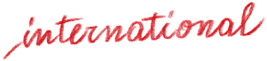# Happy Mathematics – Year Three

Worksheets, games and activities for primary school

Product: Book

ISBN: 978-88-590-1484-3

Publication date: 01/01/2018

Suitable for: Primary 2nd level (ages 8-10)

The third volume in the series, this book guides students on a mathematical learning journey divided into stages of increasing difficulty.

Several different activities are presented: 3 digit numbers, addition, subtraction, multiplication, and division (horizontal and in columns), fractions, decimal numbers, geometric shapes.  Thanks to the lively pictures and scenes featuring the characters from the De Gattis family, the children will be engaged in solving calculations and problems.

–          Numbers up to 999

–          Addition/subtraction and their properties, word problems

–          Horizontal multiplication and division, their properties, word problems

–          Multiplication and division in columns, word problems

–          Numbers up to 9999

–          Numbers up to 9999, horizontal operations

–          Numbers up to 9999, horizontal/column operations, word problems

–          Fractions, word problems

–          Decimal fractions, decimal numbers

–          Measurement

–          Measurement and problems with time

–          Geometric shapes: characteristics, elements, diagrams, probability

Thematic Areas